## Wednesday, May 16, 2012

### Simple Labs' Quick Start Kit for Arduino - Sensor Interfacing - LDR Simple Light Sensor- How To?

LDR - Light Dependent Resistor - A Simple Light Sensor
The LDR is a variable resistor, whose resistance varies based on the light incident upon it. More the light, less the resistance. Now the LDR cannot be used directly. We would need to convert the change in resistance of the ldr to change in voltage. We can achieve this by constructing a potential divider using the ldr and a fixed resistor. Then we can take the output of the potential divider and connect it to an Analog In pin on the arduino.

Follow the images below and connect the LDR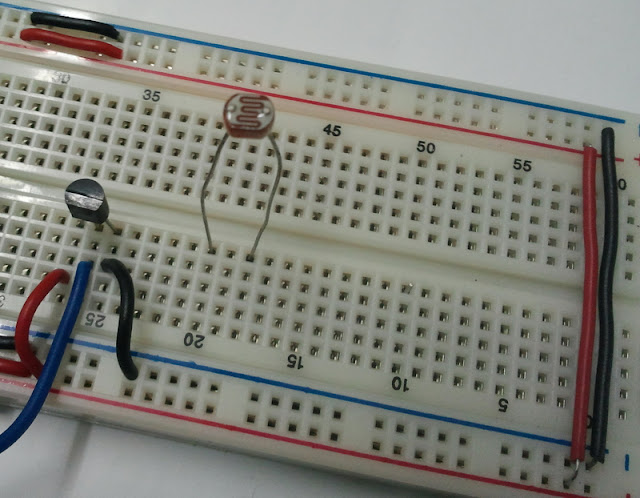This is the LDR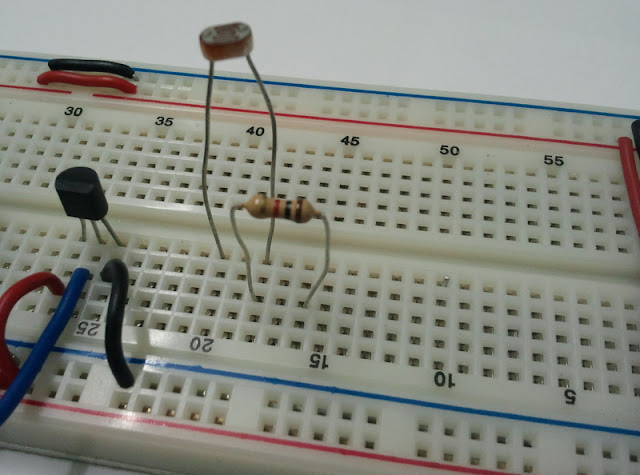Place the LDR as shown and connect a resistor as shown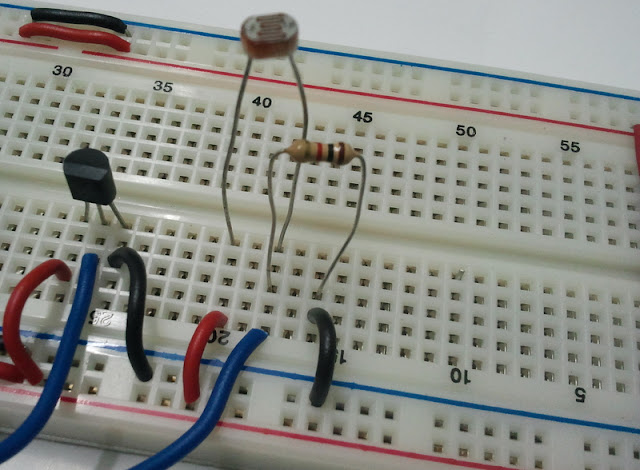Connect Supply & Ground. Take a Wire from the Junction of the Resistor & LDR and connect it to Analog In 4 of the Arduino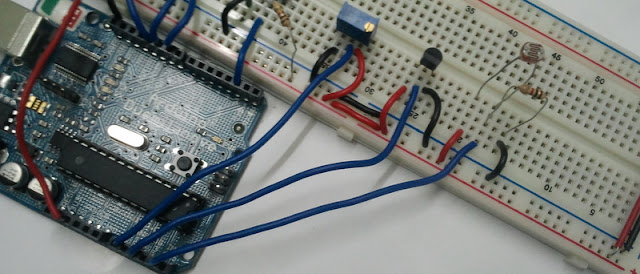Here is how the Finished Setup will look like
Try the following Code [RGB_button_ldr.ino]
```/*

Pressing the Button changes the current active color of the RGB LED & prints the current temperature value to the Serial Monitor

and the light intensity detected by the LDR determines the intensity level of the active color
*/

int intensity = 0, pin = 9;
void setup() {
pinMode(2,INPUT);
Serial.begin(9600);
}

void loop() {
if(digitalRead(2)==0) // Switch being pressed
{
int temp = analogRead(2)/2;
Serial.print("Temperature is : ");
Serial.println(temp);
analogWrite(10,0);
analogWrite(9,0);
analogWrite(11,0);
if(pin<11)
pin++;
else
pin = 9;
intensity = analogRead(4)/4;
analogWrite(pin,intensity);
Serial.print(pin);
Serial.print(" ");
Serial.println(intensity);
while(digitalRead(2)==0);
delay(100);
}

}

```

#### 3 comments:

1.Please check the spelling mistakes in program [RGB_button_ldr.ino] and it gives compiling errors. I had corrected the errors and the program is as follows:

/*
Pressing the Button changes the current active color of the RGB LED & prints the current temperature value to the Serial Monitor and the light intensity detected by the LDR determines the intensity level of the active color
*/

int intensity = 0, pin = 9;
void setup() {
pinMode(2,INPUT);
Serial.begin(9600);
}

void loop() {
if(digitalRead(2)==0) // Switch being pressed
{
Serial.print("Temperature is : ");
int temp = analogRead(2)/2;
analogWrite(10,0);

Serial.println(temp);
if(pin<11)
pin++;
else
pin=9;
analogWrite(9,0);

analogWrite(11,0);
while(digitalRead(2)==0);
delay(100);
}
intensity = analogRead(4)/4;
analogWrite(pin,intensity);

Serial.print(pin);
Serial.print(" ");
Serial.println(intensity);

}

1.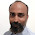Thanks a Ton... Looks like it got messed up somewhere during the code formating...

2.@Farid:
Can you guys enable "Subscribe By email" so that I can get all your posts in my Inbox, don't want to miss any posts via my Feed Reader :)

Thanks.

- Himanshu.